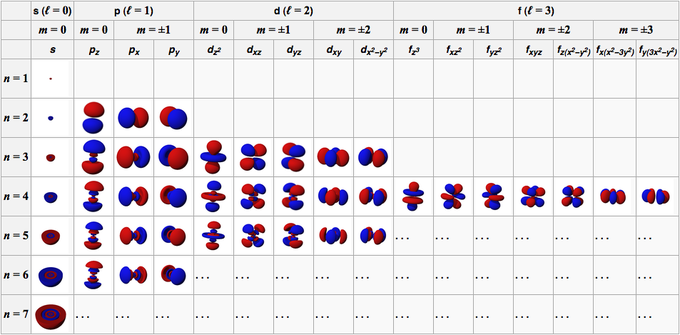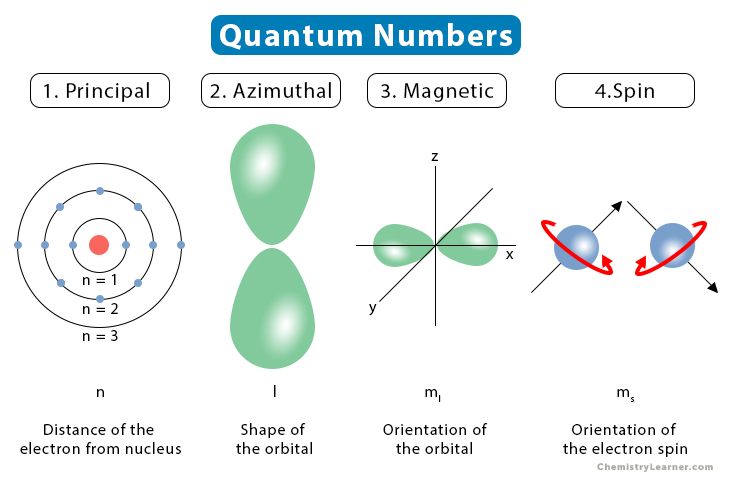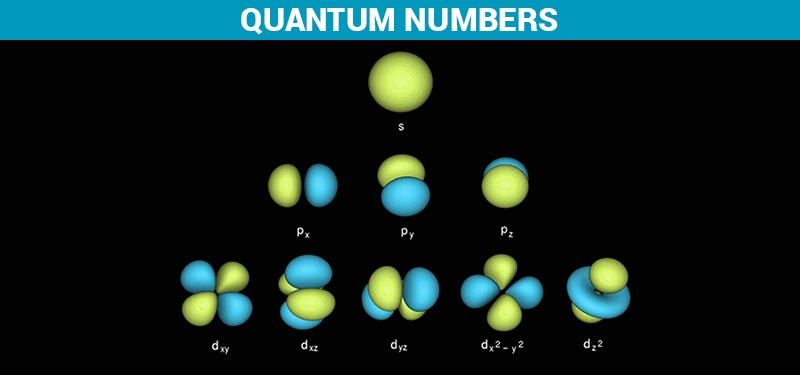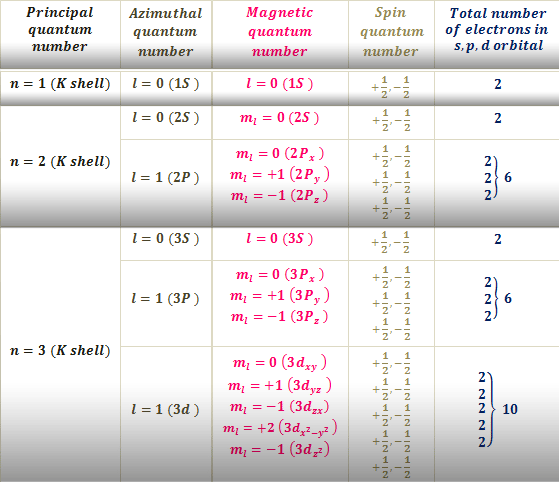# Quantum Numbers Study Guide

INTRODUCTION

Remember the last time we went to see a movie in a theater. When we booked our seats, we were probably given a ticket containing information like the show’s time, the screen number, row, and seat number. In that interval of time, you are the only person to whom that seat has been designated.Source

Similar is the case of your postal address. You have a country, state, city, locality, house number, and PIN code. These are all basically just a set of information we use to provide the exact location of our house in this big wide world. Just like that, electron quantum numbers are a set of numbers that describe the location and energy of an electron in an atom at a given moment.

### WHAT ARE QUANTUM NUMBERS?

The collection of numbers used to characterize the location and energy of an electron in an atom is known as quantum numbers. Four quantum numbers are required to fully represent an electron in an atom: principal quantum number (n), angular momentum quantum number (l), magnetic quantum number (ml), and electron spin quantum number (ms).Source

Principal Quantum Numbers

The electron shell, or energy level, of an atom, is described by principal quantum numbers, which are indicated by the sign ‘n.’ Any number with a positive value equal to or higher than one can be used as the primary quantum number’s value. The number n=1 signifies an atom’s innermost electron shell that equates to an electron’s lowest energy level.

Azimuthal Quantum Number (Orbital Angular Momentum Quantum Number)

The azimuthal quantum number for an atomic orbital describes the shape of an orbital and determines its angular momentum. Its magnitude is equivalent to the number of angular nodes within that orbital and is indicated by the symbol ‘l.’

Magnetic Quantum Number

The magnetic quantum number determines the total number of orbitals in a subshell as well as their orientation. This value represents the distribution of the orbital angular momentum along a specific axis.Source

Electron Spin Quantum Number

The values of n, l, and ml have no bearing on the electron spin quantum number. The orientation in which the electrons are spinning is determined by the value of this number. The electron spin quantum number has two potential values: 1/2 and -1/2.Source

## CONCLUSION:

• Quantum numbers are a set of numbers that describe the position and energy of an electron in an atom.
• The electron shell, or energy level, of an atom,, is described by principal quantum numbers
• The magnetic quantum number determines the total number of orbitals in a subshell as well as their orientation.

### FAQs:

1. What are the four quantum numbers?

Four quantum numbers are required to fully represent an electron in an atom: principal quantum number (n), angular momentum quantum number (l), magnetic quantum number (ml), and electron spin quantum number (ms).

2. Explain quantum numbers in chemistry and their types?

The collection of numbers used to characterize the location and energy of an electron in an atom is known as quantum numbers. Quantum numbers are divided into four categories: principal, azimuthal, magnetic, and spin. Quantum numbers are the values of the preserved quantities in a quantum system.

3. What is the spin of an electron?

Electron spin is a quantum feature of electrons. It’s a form with angular motion. As a teaching tool, teachers compare electron spin to the globe revolving by its own axis every 24 hours. Spin-up occurs when an electron spins clockwise on its axis; spin-down occurs when the electron spins anti-clockwise.

We hope you enjoyed studying this lesson and learned something cool about Quantum Numbers! Join our Discord community to get any questions you may have answered and to engage with other students just like you! Don’t forget to download our app to experience our fun VR classrooms – we promise it makes studying much more fun! 😎

## SOURCES:

1. Quantum numbers: https://www.ck12.org/c/chemistry/quantum-numbers/lesson/Quantum-Numbers-CHEM/ accessed 5 Feb 2022.
2. Different quantum numbers: https://byjus.com/chemistry/quantum-numbers/#:~:text=The%20set%20of%20numbers%20used,are%20given%20by%20quantum%20numbers. Accessed 5 Feb 2022
3. Quantum numbers: https://courses.lumenlearning.com/introchem/chapter/quantum-numbers/ Accessed 5 Feb 2022.
]]>